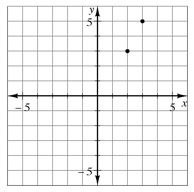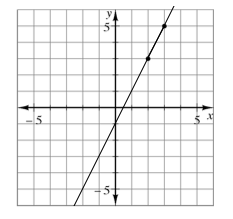### Home > MC2 > Chapter 10 > Lesson 10.2.1 > Problem10-86

10-86.

Copy the graph below on graph paper. Draw a line through the two points.1. What is the slope of the line?

Remember, slope refers to $\frac{rise}{run}$.

2. What is the rule for all points on the line?

Where is the $y$-intercept located?
How can you use this to find the rule?

$y = 2x − 1$